## Tamilnadu Samacheer Kalvi 10th Maths Solutions Chapter 1 Relations and Functions Unit Exercise 1

10th Maths Unit Exercise 1 Question 1.
If the ordered pairs (x2 – 3x, y2 + 4y) and (-2, 5) are equal, then find x and y.
Solution:
(x2 – 3x, y+ 4y) = (-2, 5)
x2 – 3x = -2
x2 – 3x + 2 = 0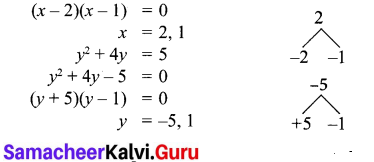10th Maths Chapter 1 Unit Exercise Question 2.
The cartesian product A × A has 9 elements among which (-1, 0) and (0,1) are found.
Find the set A and the remaining elements of A × A.
n(A × A) = 9
n(A) = 3
A = {-1,0,1}
A × A = {-1, 0, 1} × {-1, 0, 1}
A × A = {(-1,-1)(-1, 0) (-1, 1)
(0, -1) (0, 0) (0, 1)
(1,-1) (1, 0) (1, 1)}
The remaining elements of A × A =
{(-1, -1) (-1, 1) (0, -1) (0, 0) (1,-1) (1,0) (1,1)}

10th Maths Unit 1 Question 3.
Given that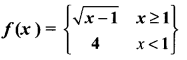(i) f(0)
(ii) f(3)
(iii) f(a + 1) in terms of a.(Given that a > 0)
Solution:
(i) f(0) = 4
(ii) f(3) = $$\sqrt{3-1}=\sqrt{2}$$
(iii) f(a + 1) = $$\sqrt{a+1-1}=\sqrt{a}$$

Samacheer Kalvi 10th Maths Book Solutions Question 4.
Let A = {9,10,11,12,13,14,15,16,17} and let f : A → N be defined by f(n) = the highest prime factor of n ∈ A. Write f as a set of ordered pairs and find the range of f.
A= {9, 10, 11, 12, 13, 14, 15, 16, 17}
f: A → N
f(x) = the highest prime factor n ∈ A
f = {(9, 3) (10, 5) (11, 11) (12, 3) (13, 13) (14, 7) (15, 5) (16, 2) (17, 17)}
Range of f = {3, 5, 11, 13, 7, 2, 17}
= {2, 3, 5, 7, 11, 13, 17}

10th Maths Exercise 1.1 Samacheer Kalvi Question 5.
Find the domain of the function f(x) = $$\sqrt{1+\sqrt{1-\sqrt{1-x^{2}}}}$$
Solution:
f(x) = $$\sqrt{1+\sqrt{1-\sqrt{1-x^{2}}}}$$
Domain of f(x) = {-1, 0, 1}
(x2 = 1, -1, 0, because $$\sqrt{1-x^{2}}$$ should be +ve, or 0)

10th Maths Unit Exercise Solutions Question 6.
If f (x) = x2, g(x) = 3x and h(x) = x – 2, Prove that (f o g)o h = f o(g o h).
f(x) = x2 ; g(x) = 3x and h(x) = x – 2
L.H.S. = (fog) oh
fog = f[g(x)]
= f(3x)
= (3x)2 = 9x2
(fog) oh = fog[h(x)]
= fog (x – 2)
= 9(x – 2)2
= 9[x2 – 4x + 4]
= 9x2 – 36x + 36 ….(1)
R.H.S. = fo(goh)
goh = g [h(x)]
= g(x – 2)
= 3(x – 2)
= 3x – 6
fo(goh) = fo [goh (x)]
= f(3x – 6)
= (3x – 6)2
= 9x2 – 36x + 36 ….(2)
From (1) and (2) we get
L.H.S. = R.H.S.
(fog) oh = fo {goh)

Samacheer Kalvi 10th Maths Exercise 1.1 Question 7.
A = {1, 2} and B = {1, 2, 3, 4} , C = {5, 6} and D = {5, 6, 7, 8} . Verify whether A × C is a subset of B × D?
Solution:
A = {1, 2), B = (1, 2, 3, 4)
C = {5, 6}, D = {5, 6, 7, 8)
A × C = {(1, 5), (1, 6), (2, 5), (2, 6)}
B × D = {(1, 5), (1, 6), (1, 7), (1, 8), (2, 5), (2, 6), (2, 7), (2, 8), (3, 5), (3, 6), (3, 7), (3, 8), (4, 5), (4, 6), (4, 7), (4, 8)}
(A × C) ⊂ (B × D) It is proved.

10th Maths Unit 1 Question Paper Question 8.
If f(x) = $$\frac{x-1}{x+1}$$, x ≠ 1 show that f(f(x)) = $$-\frac{1}{x}$$, Provided x ≠ 0.
Solution: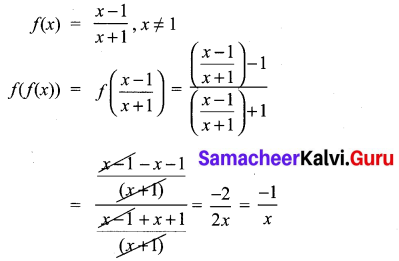Hence it is proved.

10th Maths Unit Exercise Question 9.
The function/and g are defined by f(x) = 6x + 8; g(x) = $$\frac{x-2}{3}$$.
(i) Calculate the value of $$gg\left(\frac{1}{2}\right)$$
(ii) Write an expression for g f(x) in its simplest form.
Solution: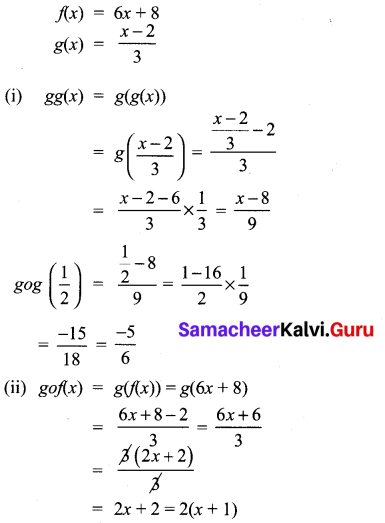10th Maths Chapter 1 Samacheer Kalvi Question 10.
Write the domain of the following real functions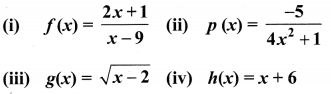Solution:
(i) f(x) = $$\frac{2x+1}{x-9}$$
The denominator should not be zero as the function is a real function.
∴ The domain = R – {9}
(ii) p(x) = $$\frac{-5}{4 x^{2}+1}$$
The domain is R.
(iii) g(x) = $$\sqrt{x-2}$$
The domain = [2, ∝)
(iv) h(x) = x + 6
The domain is R.